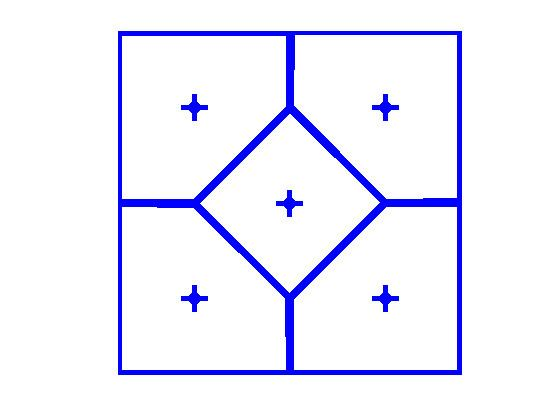# Centroidal Voronoi tessellation

Updated on
Covid-19In geometry, a centroidal Voronoi tessellation (CVT) is a special type of Voronoi tessellation or Voronoi diagram. A Voronoi tessellation is called centroidal when the generating point of each Voronoi cell is also its mean (center of mass). It can be viewed as an optimal partition corresponding to an optimal distribution of generators. A number of algorithms can be used to generate centroidal Voronoi tessellations, including Lloyd's algorithm for K-means clustering.

Gersho's conjecture, proven for one and two dimensions, says that "asymptotically speaking, all cells of the optimal CVT, while forming a tessellation, are congruent to a basic cell which depends on the dimension." In two dimensions, the basic cell for the optimal CVT is a regular hexagon.

Centroidal Voronoi tessellations are useful in data compression, optimal quadrature, optimal quantization, clustering, and optimal mesh generation. Many patterns seen in nature are closely approximated by a Centroidal Voronoi tessellation. Examples of this include the Giant's Causeway, the cells of the cornea, and the breeding pits of the male tilapia.

A weighted centroidal Voronoi diagrams is a CVT in which each centroid is weighted according to a certain function. For example, a grayscale image can be used as a density function to weight the points of a CVT, as a way to create digital stippling.

Similar Topics
Huddle (film)
Tolgahan Çoğulu
Michael Franzini
Topics